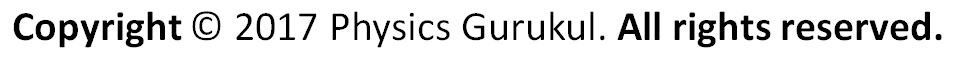# Human Eye & Colourful World (Numerical with Answer)1. A person with a myopic eye cannot see objects beyond 1.2 m distinctly. What should be the nature of corrective lens to restore proper vision? [Ans: P= -0.83 Lens – concave]2. The near point of a hypermetropic eye is at 75 cm from the eye. What is the power of the lens required to enable him to read clearly a book held at 25 cm from the eye? [Ans: P= 2.66D]3. A myopic person uses specs of power – 0.5 D. What is the distance of far point of his eye? (Ans: 2 m)4. A person wants to read a book placed at 20 cm, whereas near point of his eye is 30 cm. calculate the power of the lens required. [Ans: 1.67 D5. The far point distance of a short sighted person is 1.5 meters. find the focal length, power and nature of the remedial lens? (Ans: -1.5 m, -0.67 D, concave lens)6. A person having a myopic eye uses a concave lens of focal length 10 cm. Find the power of the lens. (Ans: -10 D)7. A person with myopic eye cannot see objects beyond 1.2 m distinctly. What should be the nature of corrective lenses to restore proper vision?Ans: Here, distance of far point, x = 1.2 m

For viewing distant objects, focal length of corrective lens, F = -x = -1.2 m,

P = 1 / f = 1 / -1.2 = -0.83D8. The far point of a myopic person is 80 cm in front of the eye. What is the nature and power of the lens required to correct the problem?Ans: For diagram, Here, x’ = 1 m = 100 cm, d = 25 cm, f =?

From f = x’d / x’- d, F = 100*25 / 100 – 25 = 33.3 cm, P = 100 / f = 100 / 33.3 = 3 D9. The far point of myopic person is 80 cm in front of the eye. What is the nature and power of the lens required to enable him to see very distant objects distinctly? [Ans: Concave; -1.25 D]10. The far point of a myopic person is 150 cm in front the eye. Calculate the focal length and power of a lens required to enable him to see distant objects clearly. [Ans: -1.5 m, -0.67 D]